AVL 平衡二叉树

## 1、为什么要有AVL平衡二叉树

我们之前写过文章叫做BST二分搜索树，这种数据结构有一个缺点就是会退化为链表形式，到这导致了我们的树结构发挥不出来它应有的优势。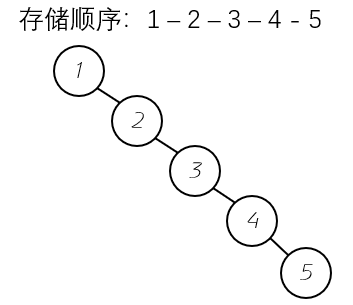从上图可以发现如果按照顺序进行添加操作，那么二分搜索树就会退化为链表形式，树结构也就失去了它的意义。

## 2、什么是AVL平衡二叉树

我们之前写过的满二叉树、完全二叉树、线段树、最大堆等等都是一种平衡树的例子（叶子节点的高度差不会大于 1 ）。其实上面的平衡二叉树都是比较理想的例子。但是在AVL树中维护的平衡二叉树有所不同。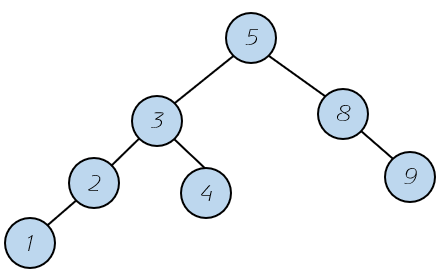这个结构并不满足我们之前的平衡二叉树的规则，根节点的左右子树高度差不大于 1 。却满足我们上面的规则（对于任意节点）。

对于时间复杂度方面，平衡二叉树的高度和节点数量之间也是O(log (N) )。

## 3、AVL树的基本实现

### 3.1、实现的方法

首先，我们需要标注每个节点的高度信息平衡因子，平衡因子的计算就是左子树的高度减去右子树的高度的绝对值。这样我们就可以依靠平衡因子来维护的AVL树结构。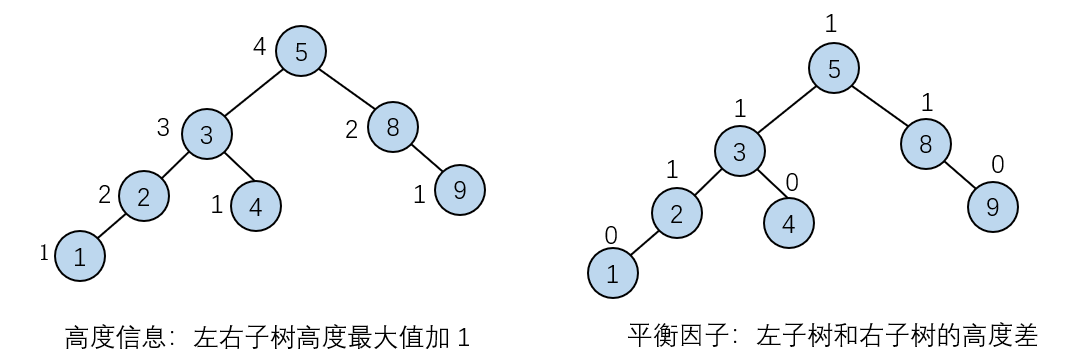### 3.2、构造函数

我们首先需要构造一个节点信息作为内部类，主要包含我们要存储的内容，左右子树的指针，还有高度信息。对于平衡因子我们可以使用左右子树的差来进行计算。

private class Node{  //内部类
public K key;
public V value;
public Node left, right;
public int height;

public Node(K key, V value){
this.key = key;
this.value = value;
left = null;
right = null;
height = 1; //新节点高度为 1
}


private Node root;
private int size;

public AVLTree(){
root = null;
size = 0;
}


### 3.3、基本成员函数

首先我们需要获取树结构的大小信息getSize()方法和判断是否为空isEmpty()方法。

public int getSize() {
return size;
}

public boolean isEmpty() {
return size == 0;
}


因为我们需要高度信息来判断树结构，所以引入getHeight()方法。

private int getHeight(Node node) {
if (node == null)
return 0;
return node.height;
}


为了方便我们后续的操作，我们引入getNode()方法，通过索引key值来获得节点。

private Node getNode(K key) {
return getNode(root, key);
}

private Node getNode(Node node, K key) {
if (node == null)
return null;
if (node.key.compareTo(key) < 0)
return getNode(node.left, key);
else if (node.key.compareTo(key) > 0)
return getNode(node.right, key);
else
return node;
}


在添加操作的时候，我们需要根据高度信息来获得平衡因子，进而判断树结构是否满足平衡树的性质。

private int getBalanceFactor(Node node){
if (node == null)
return 0;
return getHeight(node.left) - getHeight(node.right);
}


## 4、左旋转和右旋转

我们知道原来平衡的树变成不平衡会是在添加元素的时候，所以在添加元素的时候我们需要维护平衡性。下面就分四种情况分别讨论：

### 4.1、LL 右旋转

有一种添加元素后的情况是这样的。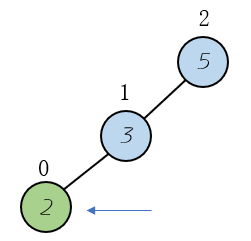可以认为添加的元素在节点的左边（L）的右边（L）。
在添加元素2，后会导致树结构的平衡性破坏，对于这种情况的判断就是当前节点的平衡因子大于1（向左偏斜），并且左节点的平衡因子大于0（向左偏斜），这样就保证了这种情况的出现，向左偏斜。

if (balanceFactor > 1 && getBalanceFactor(node.left) >= 0)

那么我们就需要对上面的结构进行维护。进行右旋转操作。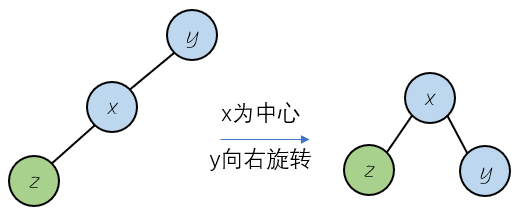为了不失一般性，我们引入更复杂的情况，如下图：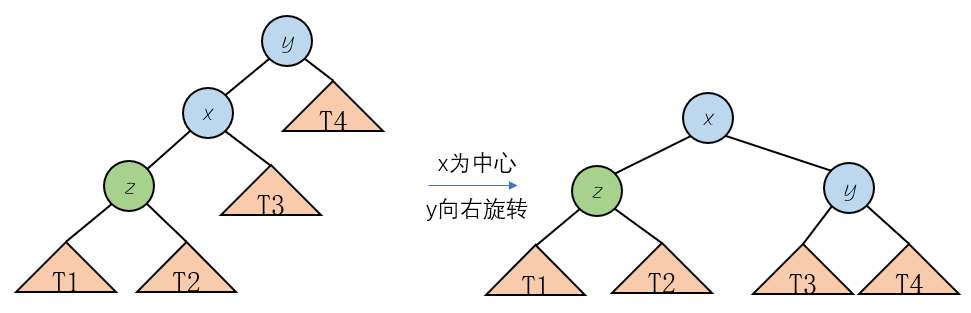按照平衡二叉树的排序规则，对元素进行右旋转（类似于 y 绕 x 右旋转），相当于降低树的高度。旋转后仍然满足二叉树的排序规则。我们可以发现相对位置发生改变的就是节点 y 和 x 的右子树 T3 。最后更新高度信息，高度发生变化的只有节点 x , y 。

private Node rightRotate(Node y) {
Node x = y.left; //获得旋转中心
Node T3 = x.right;

x.right = y;  //进行旋转操作
y.left = T3;

y.height = Math.max(getHeight(y.left), getHeight(y.right)) + 1;  //重新更新高度信息
x.height = Math.max(getHeight(x.left), getHeight(x.right)) + 1;

return x;
}


### 4.2、RR 左旋转

我们有了上面的右旋转的概念，那么左旋转就变得简单多了，左旋转的发生的前提就是节点的平衡因子小于-1（偏右），右子树的平衡因子小于0（偏右），也就是类似于下面的结构。
可以认为添加的元素在节点的右边（R）的右边（R）。

if (balanceFactor < -1 && getBalanceFactor(node.right) <= 0)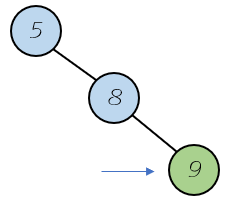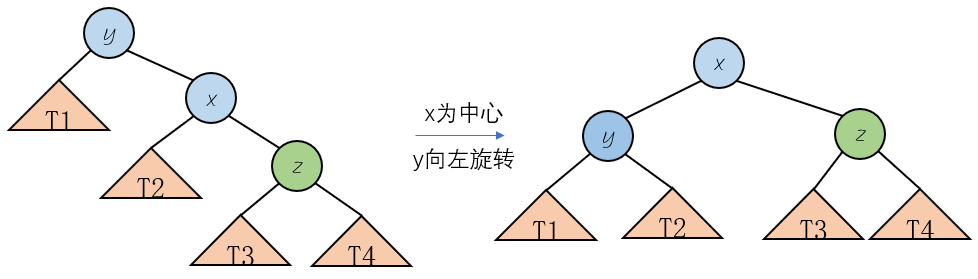以x为中心，进行左旋转操作（类似于 y 绕 x 左旋转），需要转移的分别是 x 的左子树 T2 和节点 y 。

private Node leftRotate(Node y) {

Node x = y.right;
Node T2 = x.left;

x.left = y; //需要转移的元素
y.right = T2;

y.height = Math.max(getHeight(y.left), getHeight(y.right)) + 1; // 更新高度信息
x.height = Math.max(getHeight(x.left), getHeight(x.right)) + 1;

return x;
}


### 4.3、LR 左右旋转

这种情况就是添加元素在节点的左边的右边，左右旋转指的是先进行左旋转，后进行右旋转。类似于下面这种情况。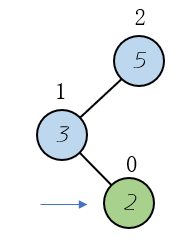先进行左旋转操作，结构就会变成我们之前LL的形式，然后对其进行右旋转操作即可。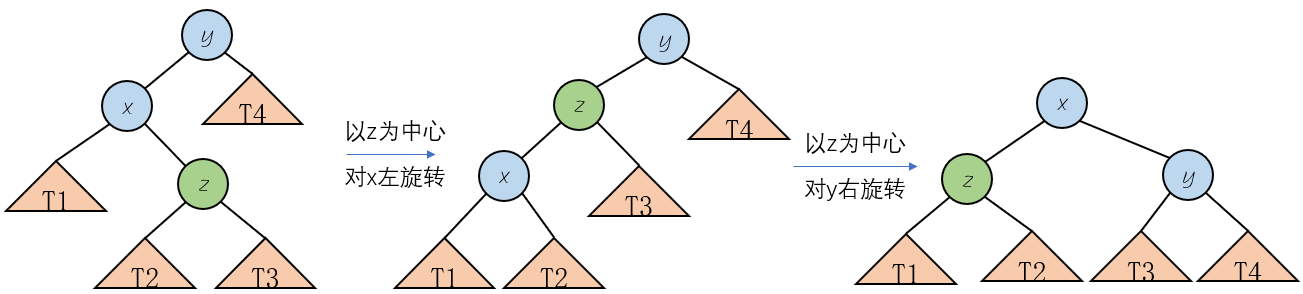### 4.4、RL 右左旋转

这种情况就是添加元素在节点的右边的左边，右左旋转指的是先进行右旋转，后进行左旋转。类似于下面这种情况。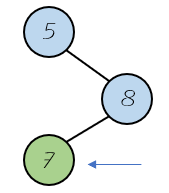先进行左旋转操作，结构就会变成我们之前LL的形式，然后对其进行右旋转操作即可。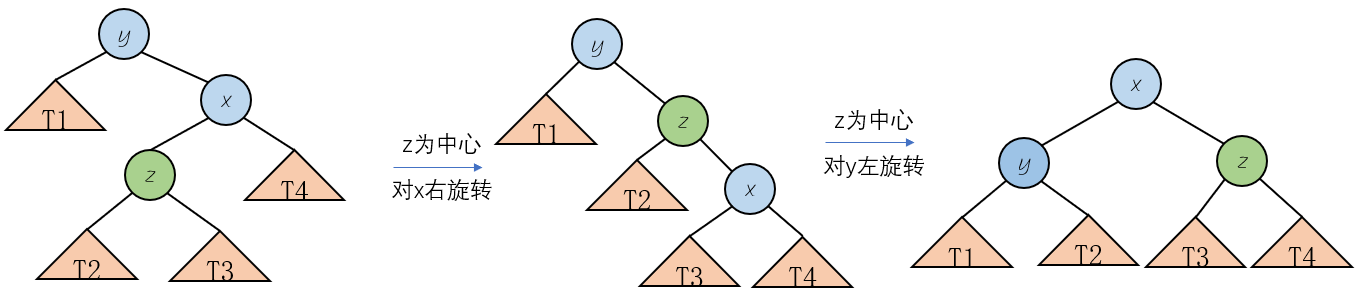### 4.5、四种情况总结

上面的四种情况完全包含了添加元素所需要的情况。

// LL
if (balanceFactor > 1 && getBalanceFactor(node.left) >= 0)
return rightRotate(node);
// RR
if (balanceFactor < -1 && getBalanceFactor(node.right) <= 0)
return leftRotate(node);
// LR
if (balanceFactor > 1 && getBalanceFactor(node.left) < 0){
node.left = leftRotate(node.left);
return rightRotate(node);
}
// RL
if (balanceFactor < -1 && getBalanceFactor(node.right) > 0){
node.right = rightRotate(node.right);
return leftRotate(node);
}


## 5、增删改查操作的实现

### 5.1、添加操纵

• 递归到底的时候添加元素，否则就更新元素
• 更新每个节点的高度信息
• 对结构进行平衡处理
private Node add(Node node, K key, V value) {
/*
* BST 的源代码片段 ····
*/
node.height = 1 + Math.max(getHeight(node.left), getHeight(node.right));
int balanceFactor = getBalanceFactor(node);
// LL
if (balanceFactor > 1 && getBalanceFactor(node.left) >= 0)
return rightRotate(node);
// RR
if (balanceFactor < -1 && getBalanceFactor(node.right) <= 0)
return leftRotate(node);
// LR
if (balanceFactor > 1 && getBalanceFactor(node.left) < 0){
node.left = leftRotate(node.left);
return rightRotate(node);
}
// RL
if (balanceFactor < -1 && getBalanceFactor(node.right) > 0){
node.right = rightRotate(node.right);
return leftRotate(node);
}
return node;
}


### 5.2、删除操作

这里的删除操作，在原本BST二分搜索树的基础上进行改变，增加删除元素后对节点进行平衡后的处理，同添加操作基本相同，这里只对增加的程序片段进行展示。


private Node remove(Node node, K key) {
/*
* BST 的源代码片段 ····
*/
if (retNode == null)
return null;
retNode.height = 1 + Math.max(getHeight(retNode.left), getHeight(retNode.right)); //更新高度值
int balanceFactor = getBalanceFactor(retNode);
// LL
if (balanceFactor > 1 && getBalanceFactor(retNode.left) >= 0)
return rightRotate(retNode);
// RR
if (balanceFactor < -1 && getBalanceFactor(retNode.right) <= 0)
return leftRotate(retNode);
// LR
if (balanceFactor > 1 && getBalanceFactor(retNode.left) < 0){
retNode.left = leftRotate(retNode.left);
return rightRotate(retNode);
}
// RL
if (balanceFactor < -1 && getBalanceFactor(retNode.right) > 0){
retNode.right = rightRotate(retNode.right);
return leftRotate(retNode);
}
return retNode;
}


### 5.3、查询操作

查询操作主要包含查看元素是否在树结构中，另一个就是通过key值查找对应的 value 值。两者均是借助getNode方法进行实现。

public boolean contains(K key) {
return getNode(key) != null;
}

public V get(K key) {
Node node = getNode(key);
return node == null ? null : node.value;
}


### 5.4、更改操作

更改操作也是借助getNode方法进行更改。

public void set(K key, V newValue) {
Node node = getNode(key);
if (node != null)
node.value = newValue;
else
throw new IllegalArgumentException(key + "doesn't exist");
}